# ICSE Selina Solution for Class 9 Chemistry Chapter 6

## ICSE Selina Solution for Class 9 Chemistry Chapter 6 PDF

ICSE Selina Solution for Class 9 Chemistry Chapter 6 provided here for the benefit of the students studying ICSE class 9 chemistry. This study material comprises answers to all the questions provided in Selina publishers ICSE Class 9 Chemistry. All the answers provided here are solved by expert teachers and will provide you with the necessary practice of solving a variety of questions on the chapter Study of Gas Laws.

ICSE Selina solution for class 9 has a different type of questions which will give you necessary practice before you face the ICSE class 9 examination. All the answers provided here are prepared as per the latest ICSE Syllabus. Exercise questions provided here has a variety of questions that will help you in getting the practice of solving question before writing class 9 examination.

ICSE Concise solutions for class 9 chemistry provide deeper insights into the position of Hydrogen in the periodic table. After studying this study material students will acquire knowledge of Hydrogen from water, displacement of hydrogen from alkalis, preparation and collection of Hydrogen, Industrial manufacture of Hydrogen by Bosch process, oxidation, reduction reaction, and their importance.

### Download PDF of ICSE Selina Solution for Class 9 Chemistry Chapter 6 The first element Hydrogen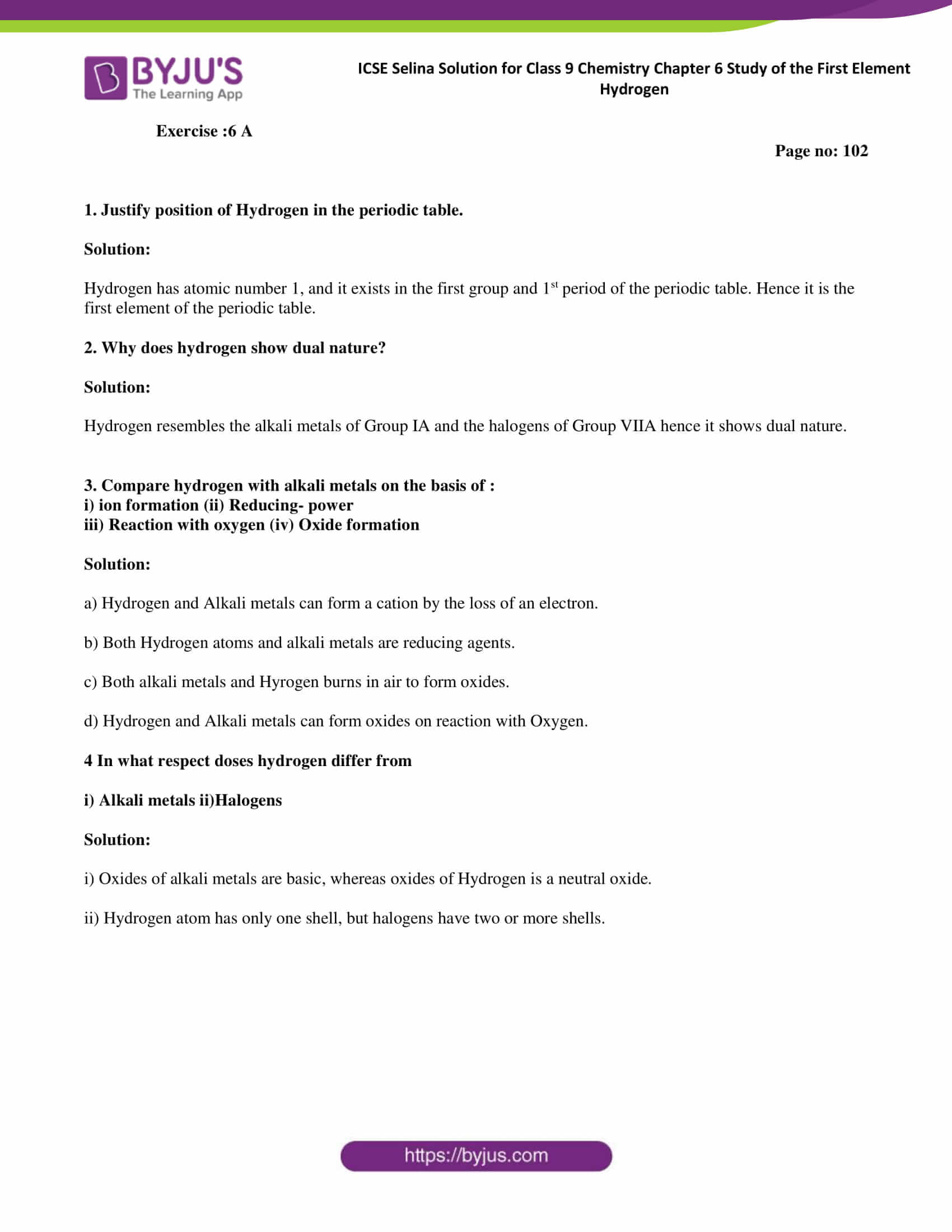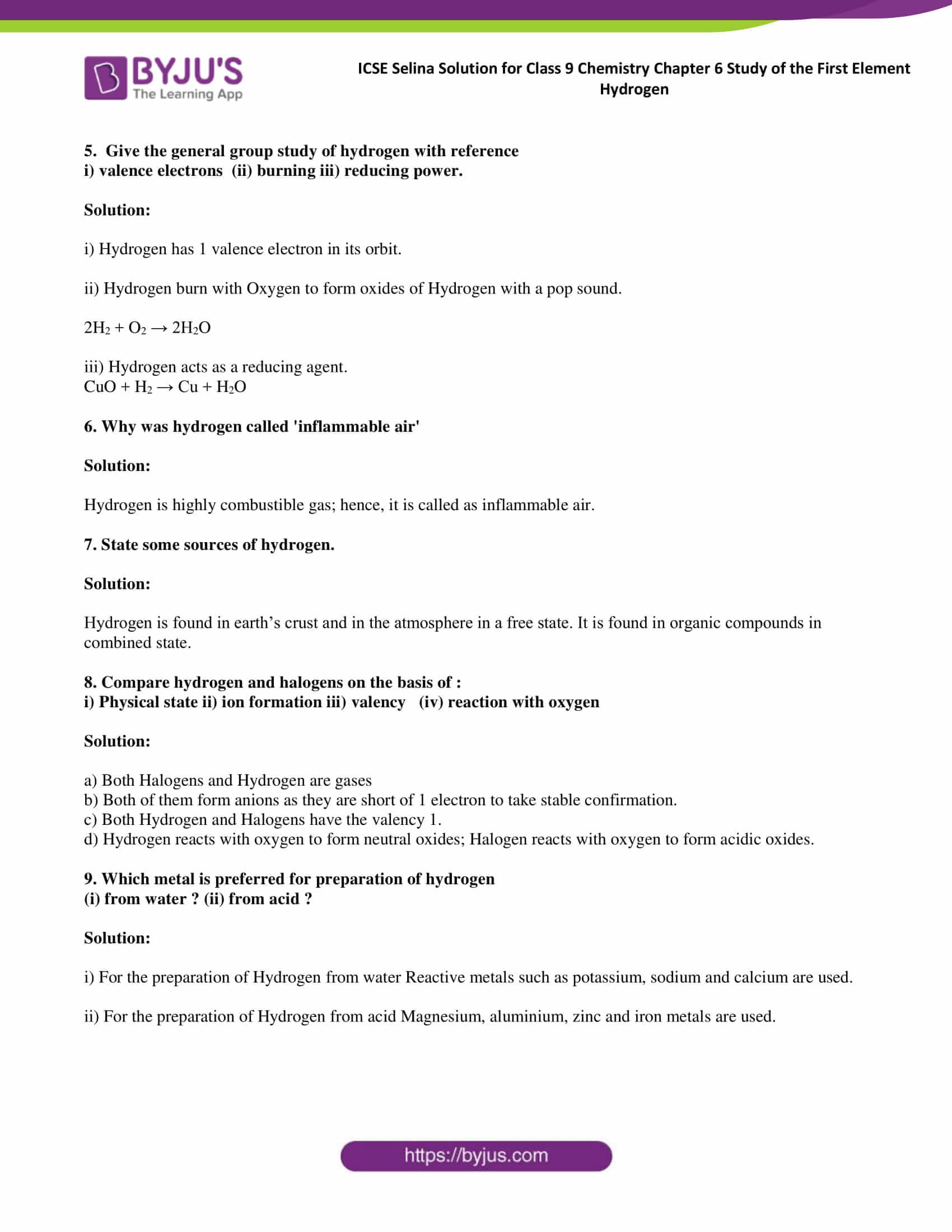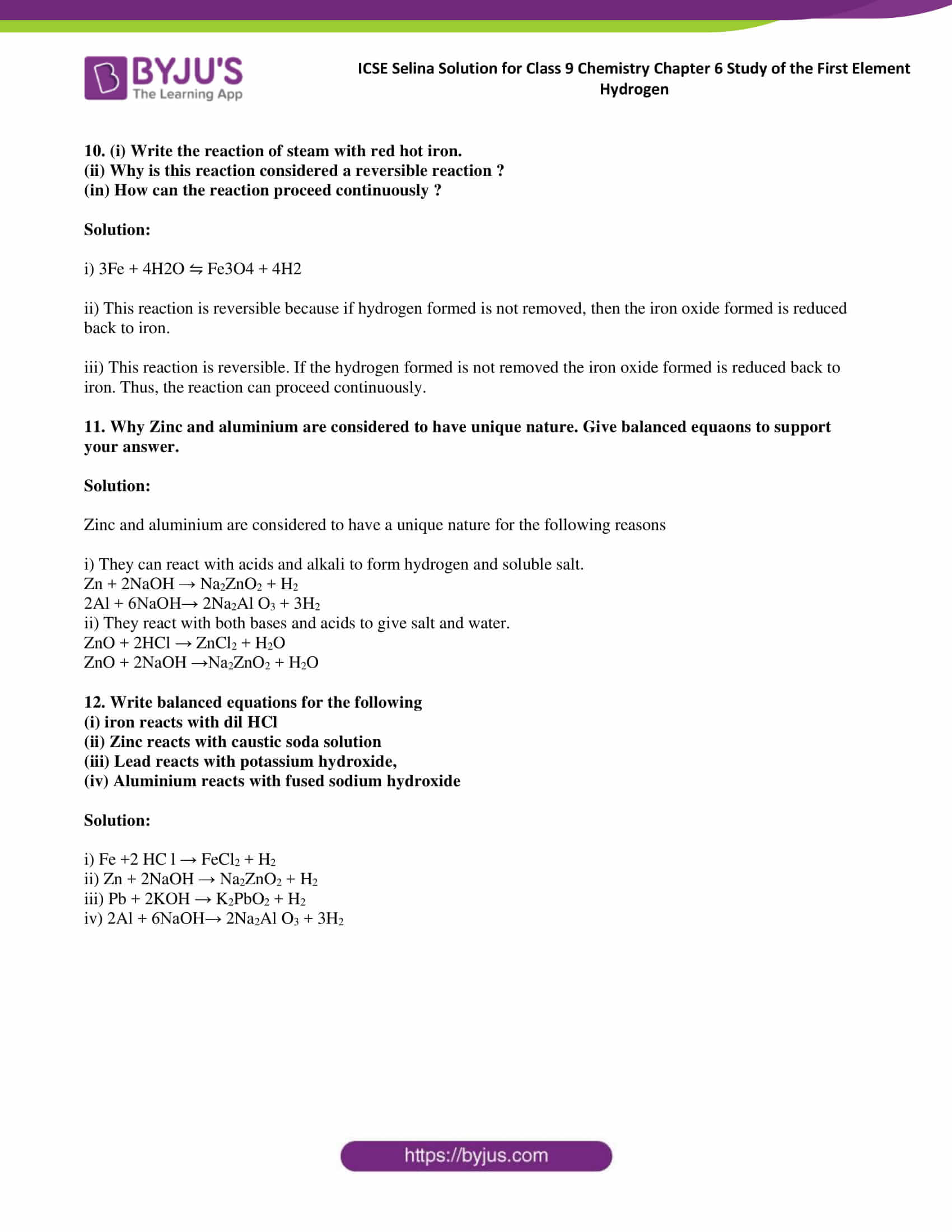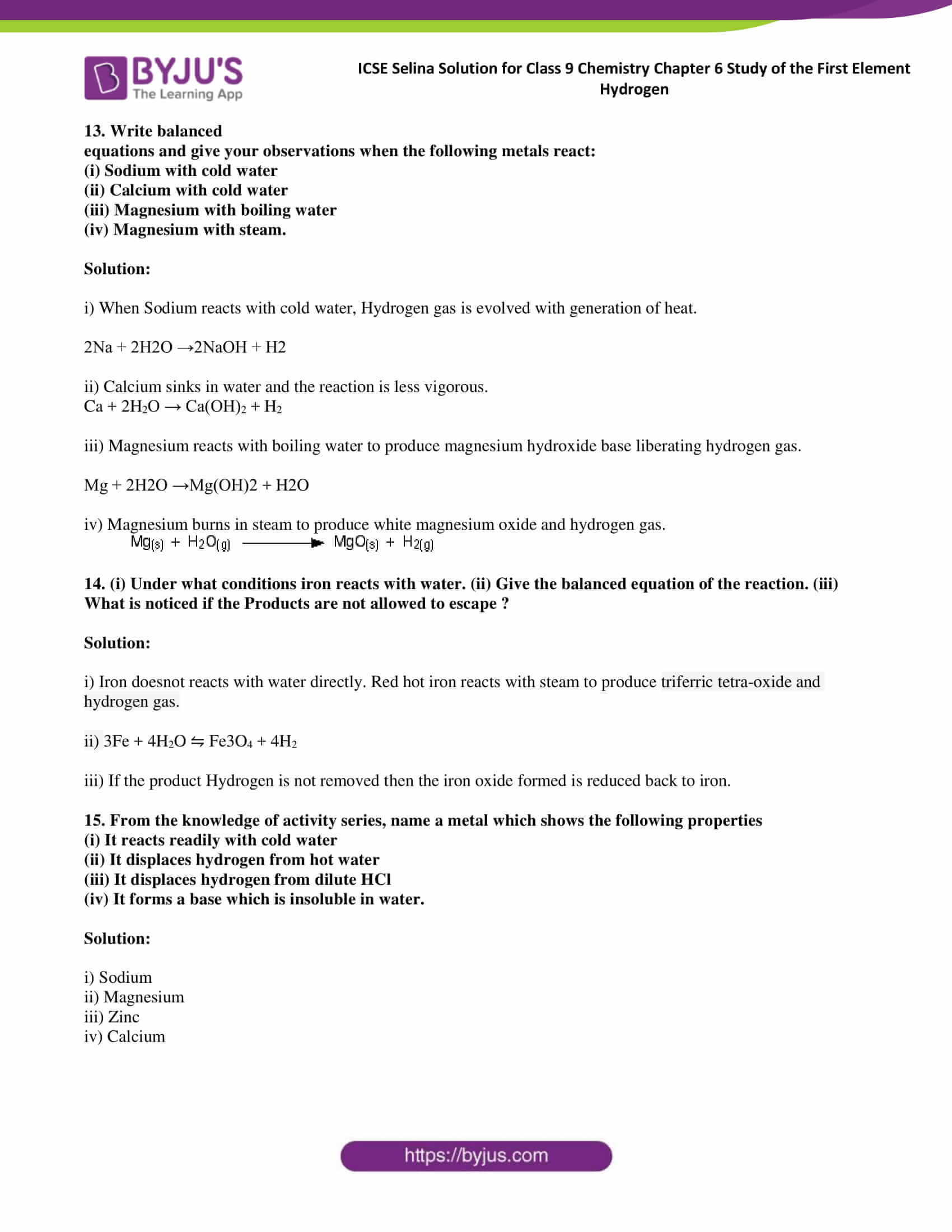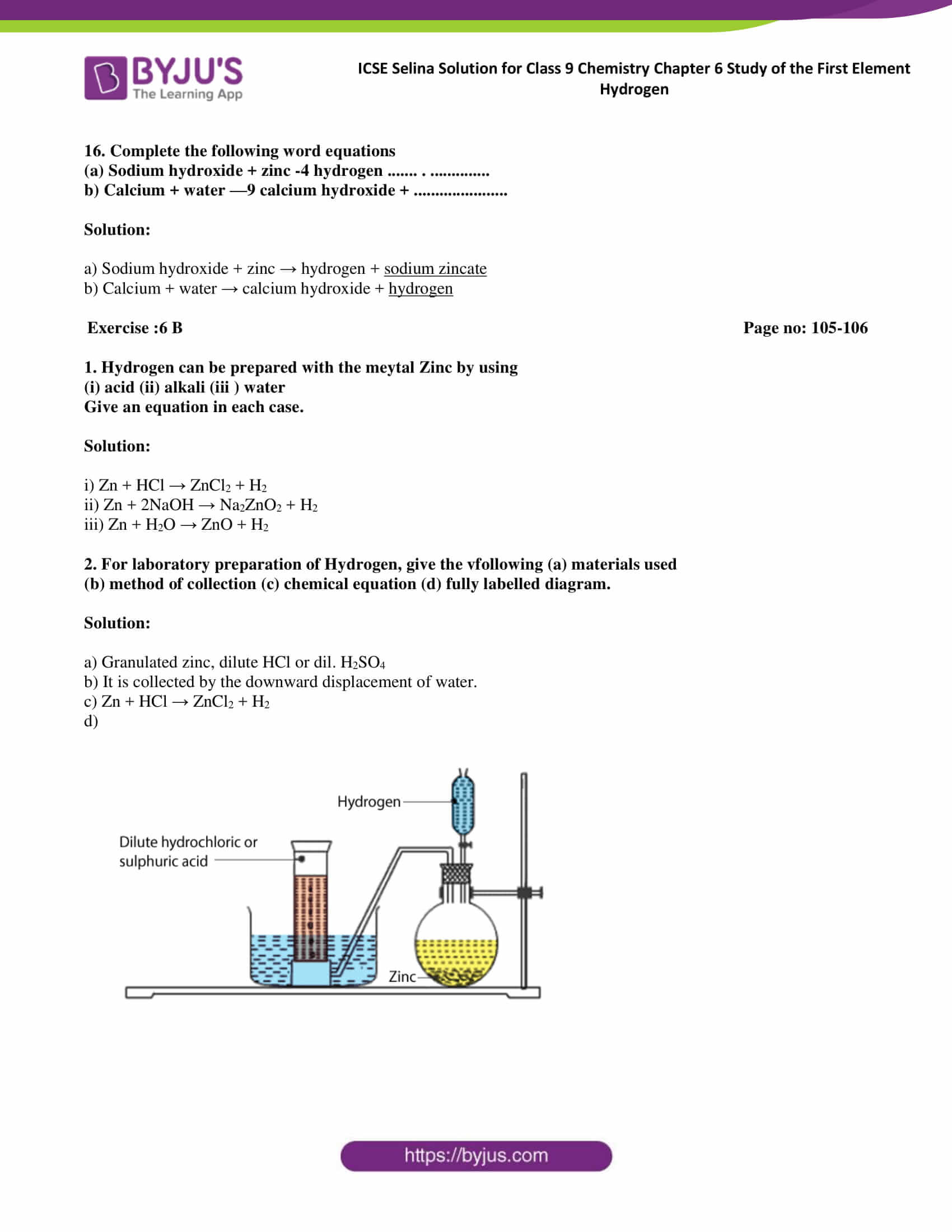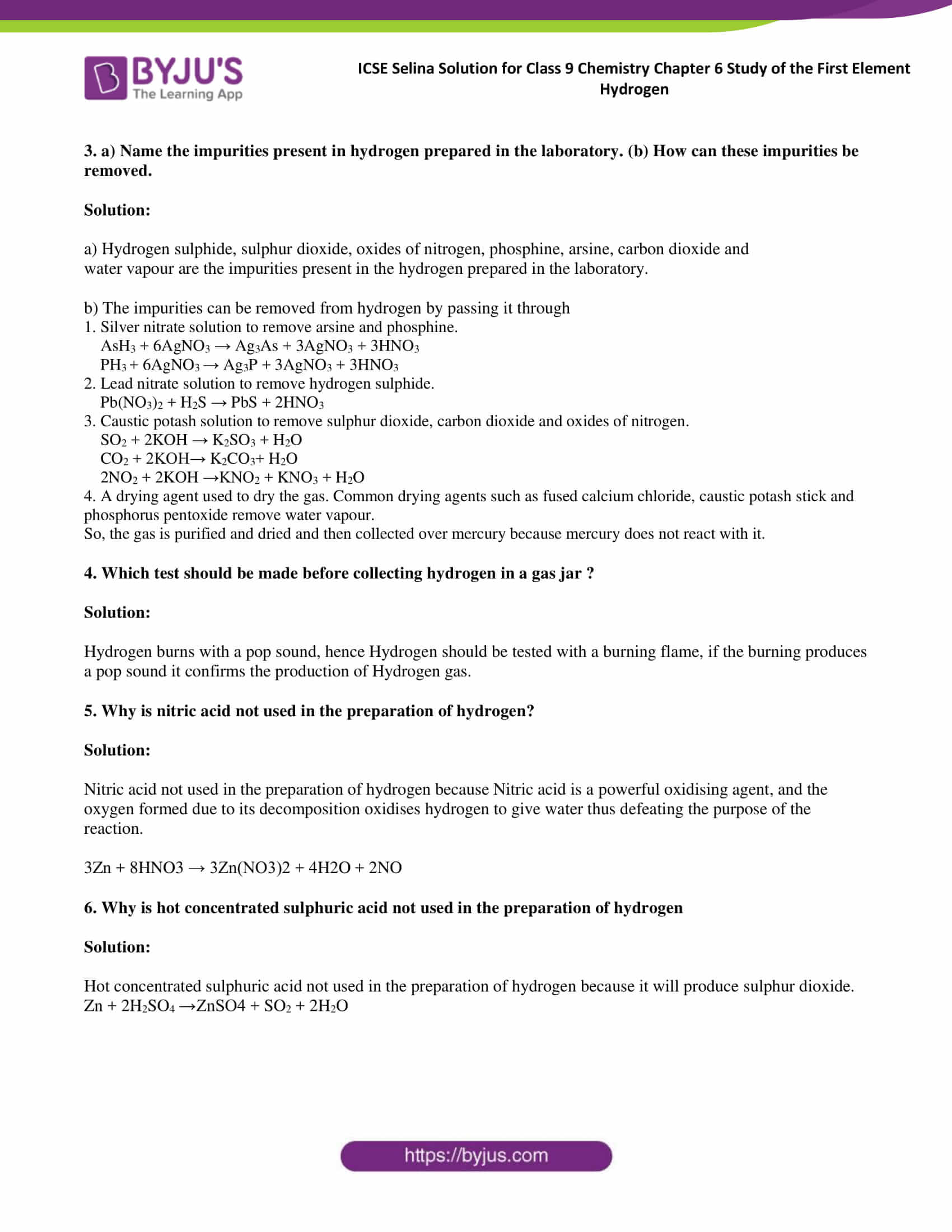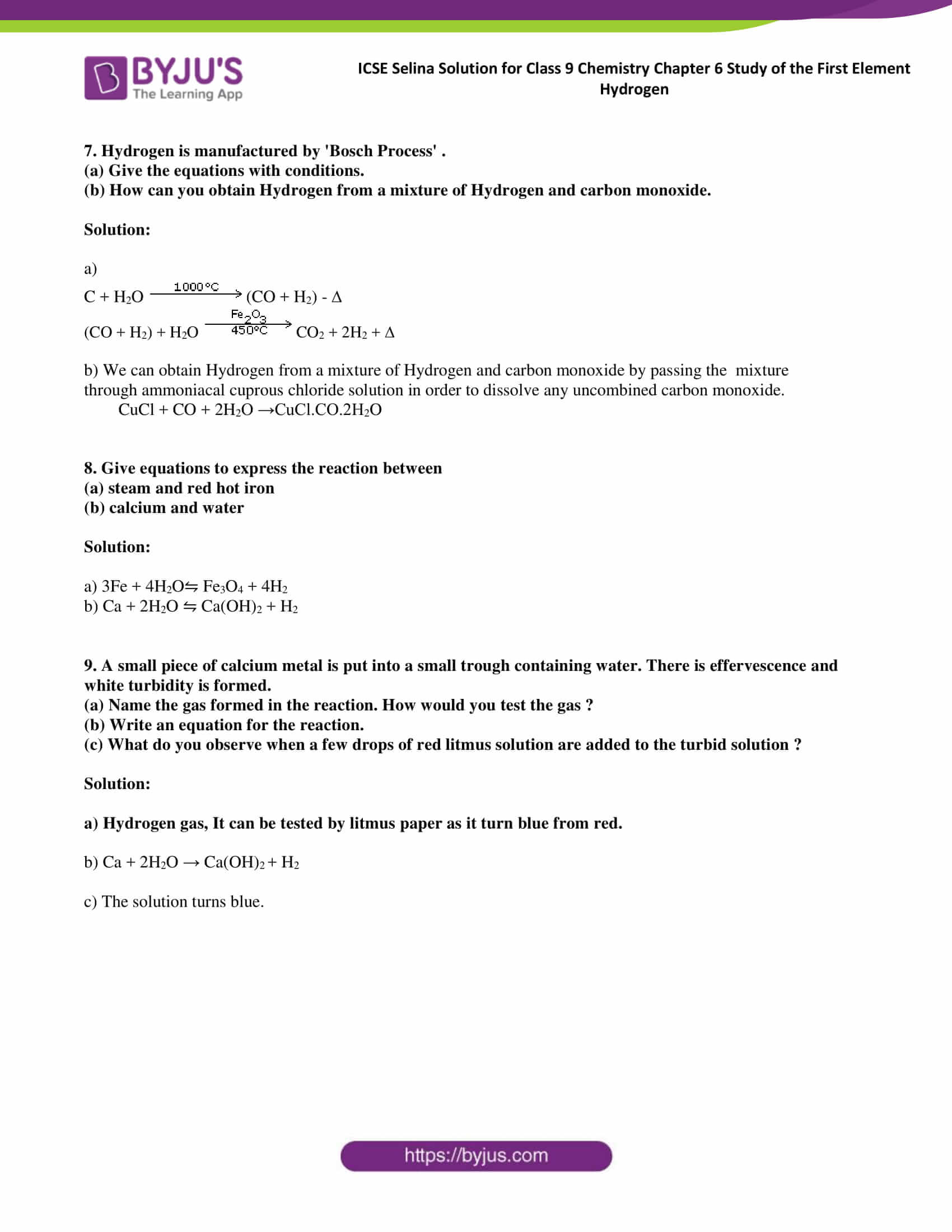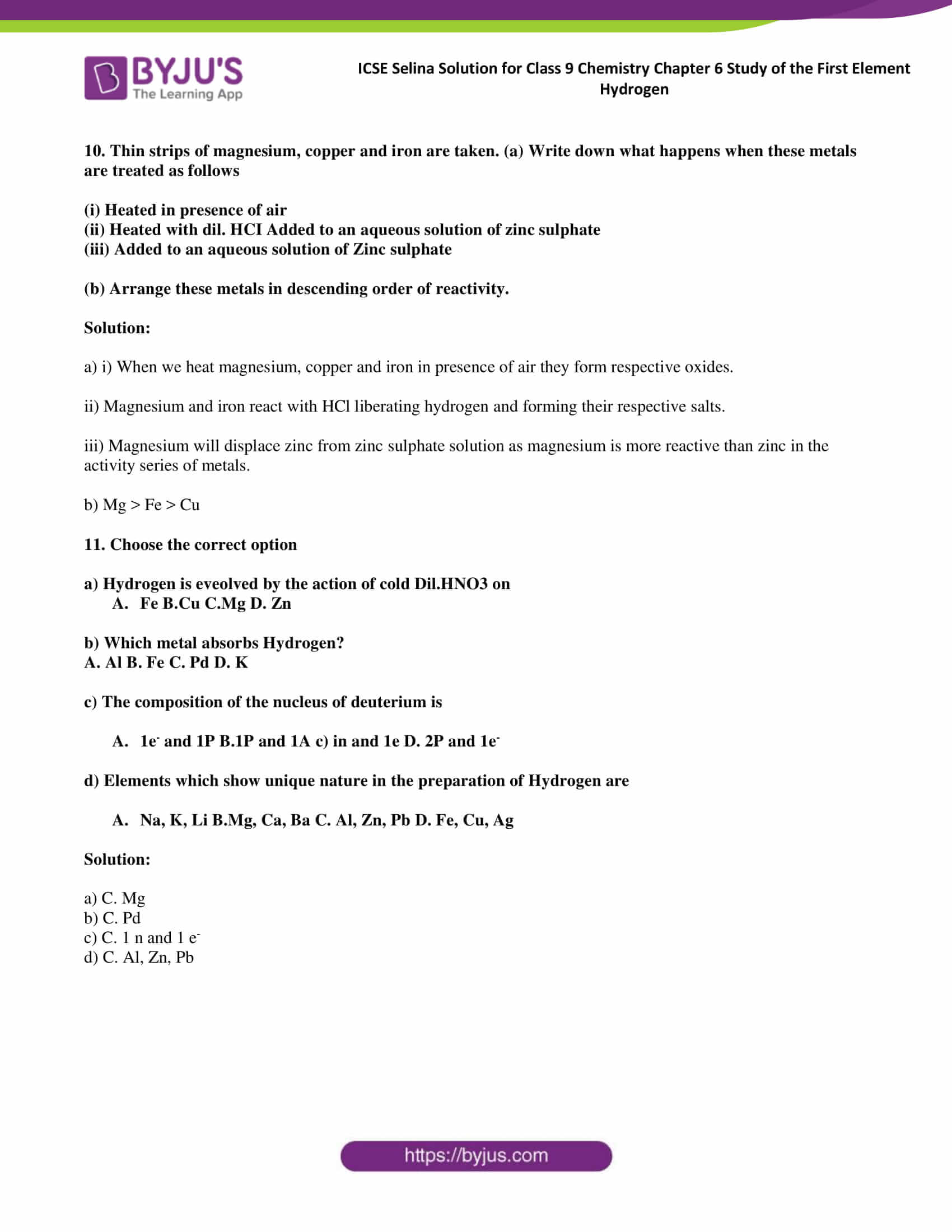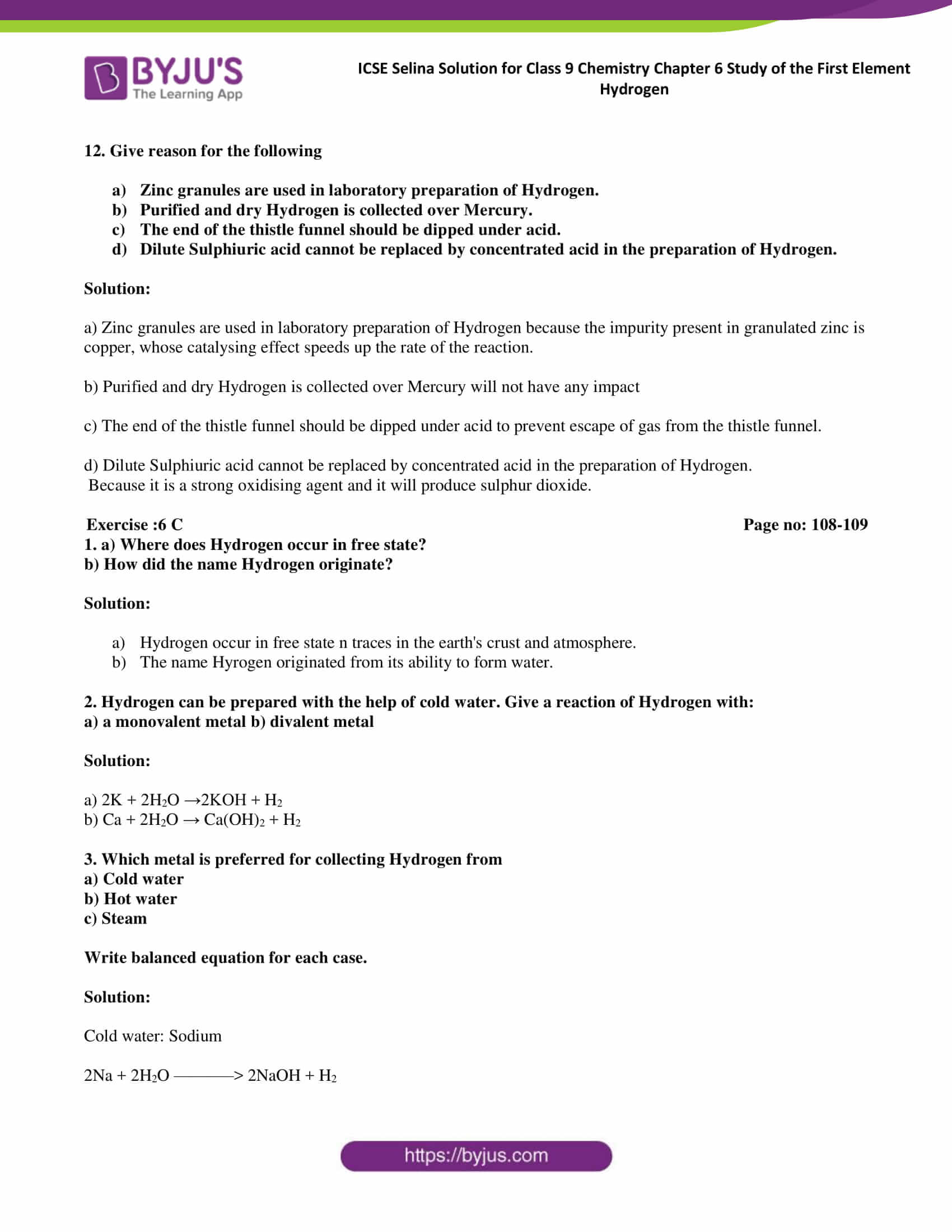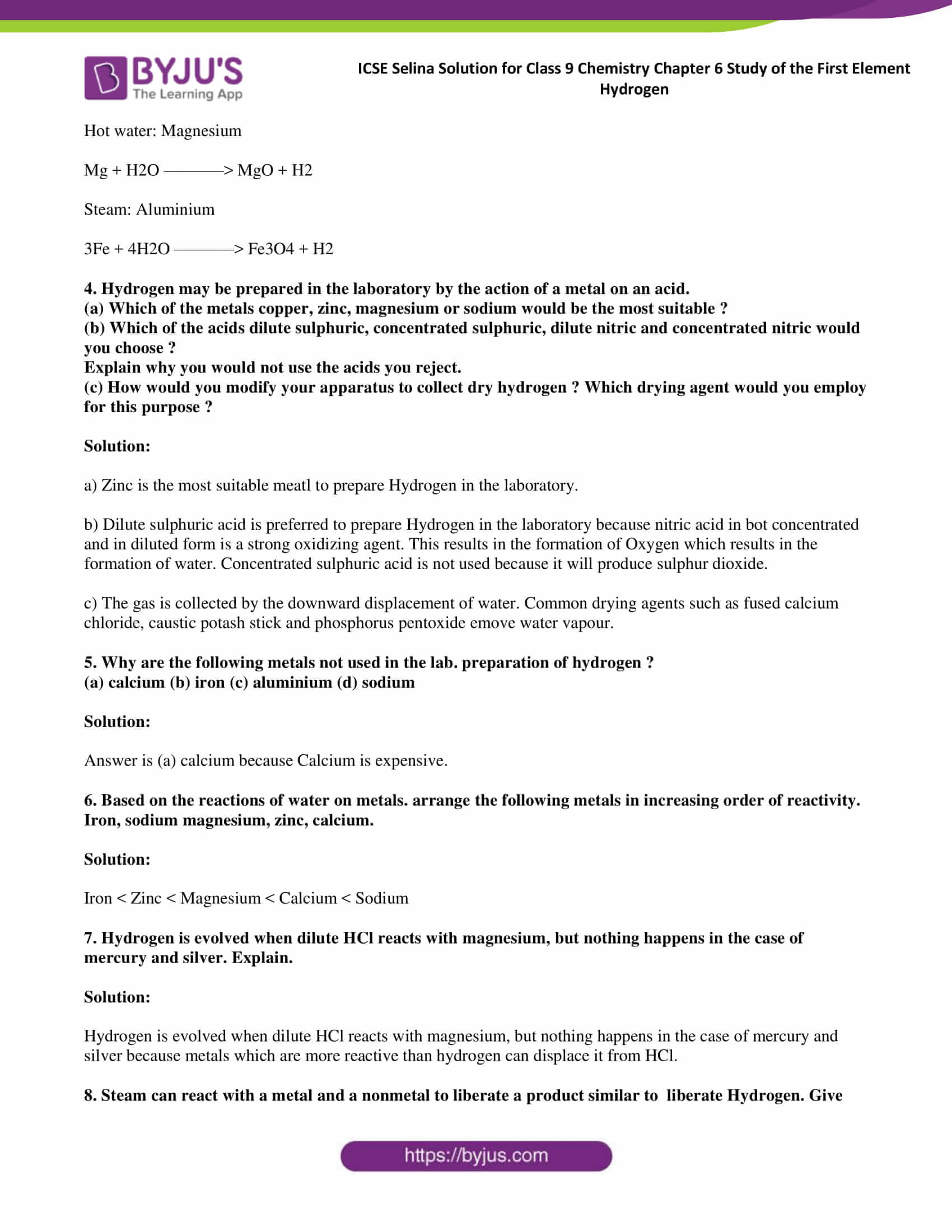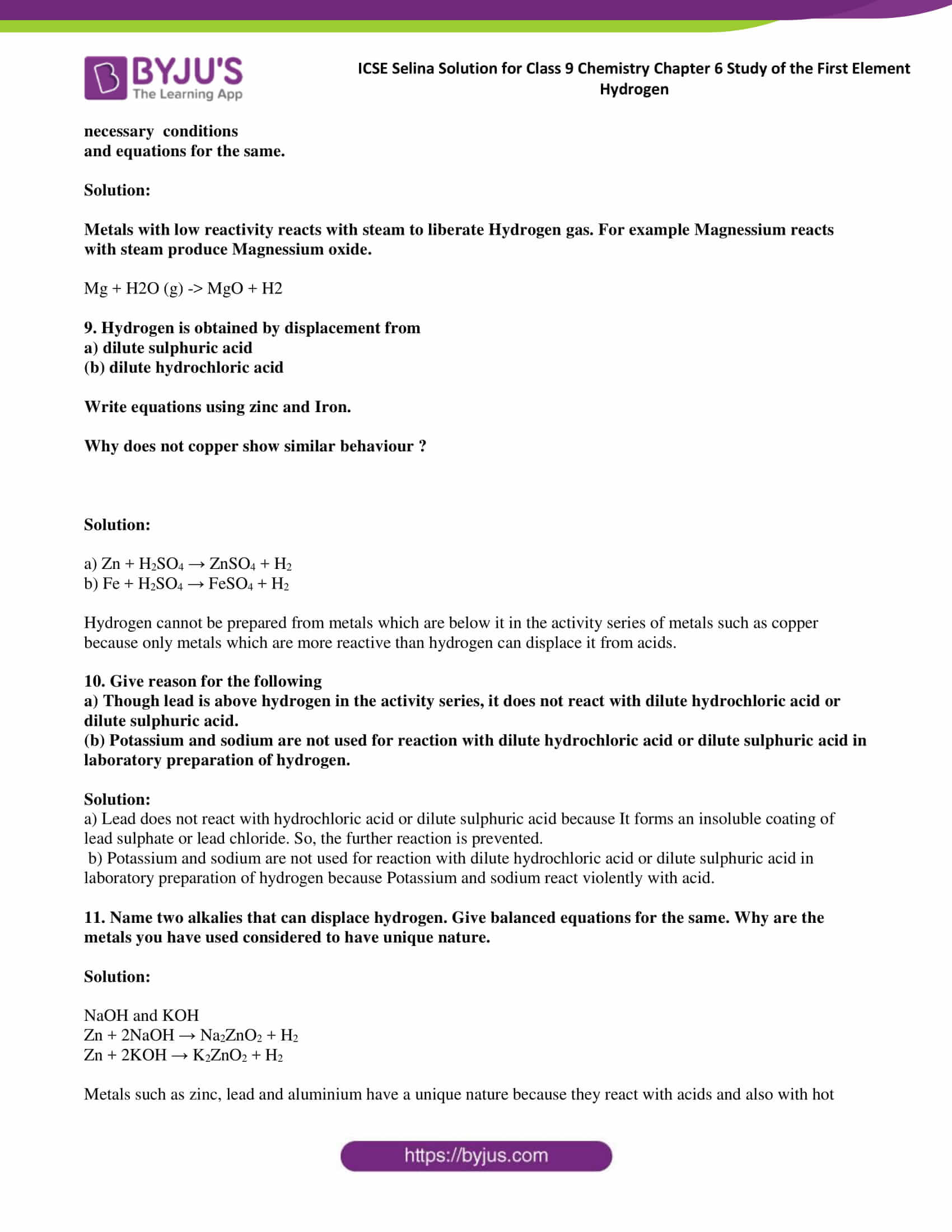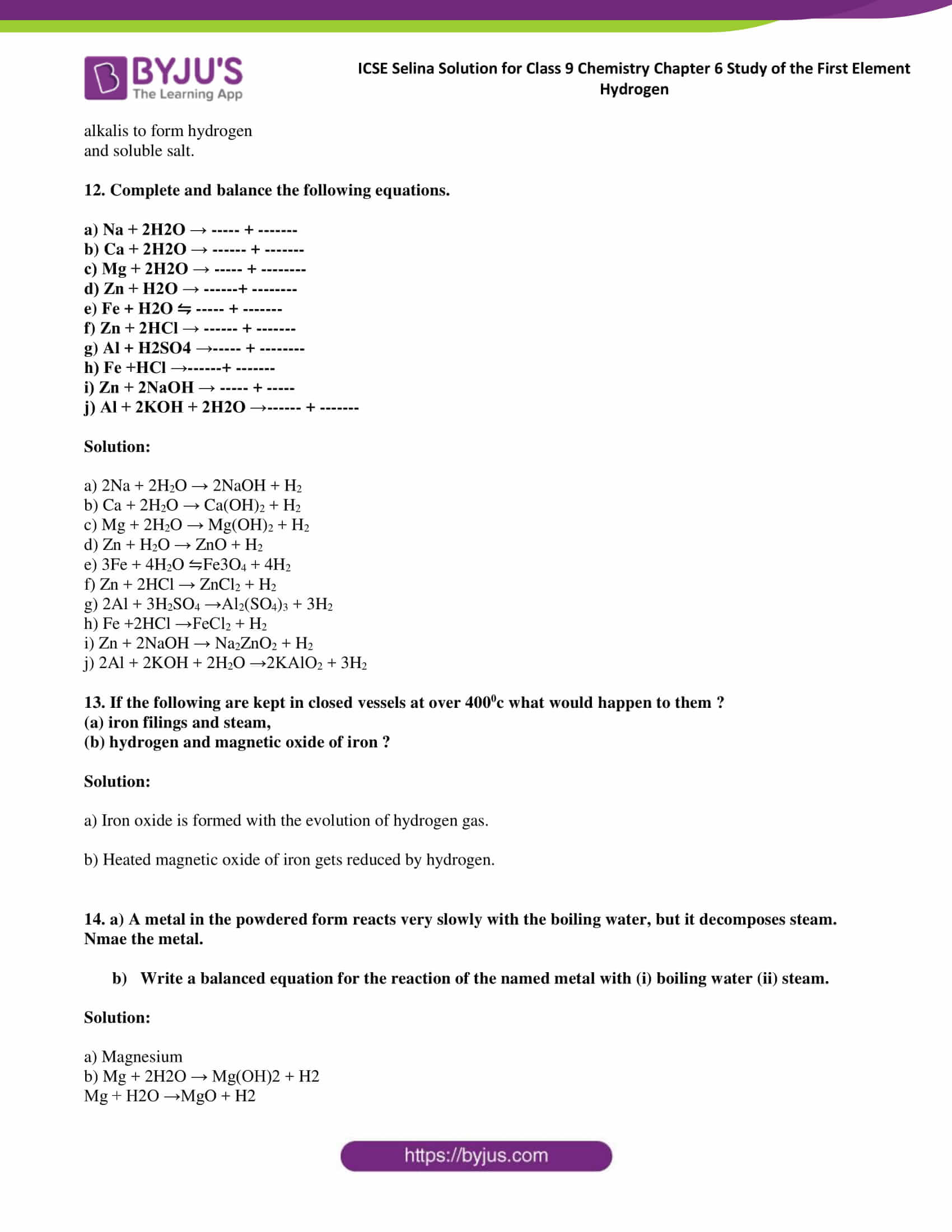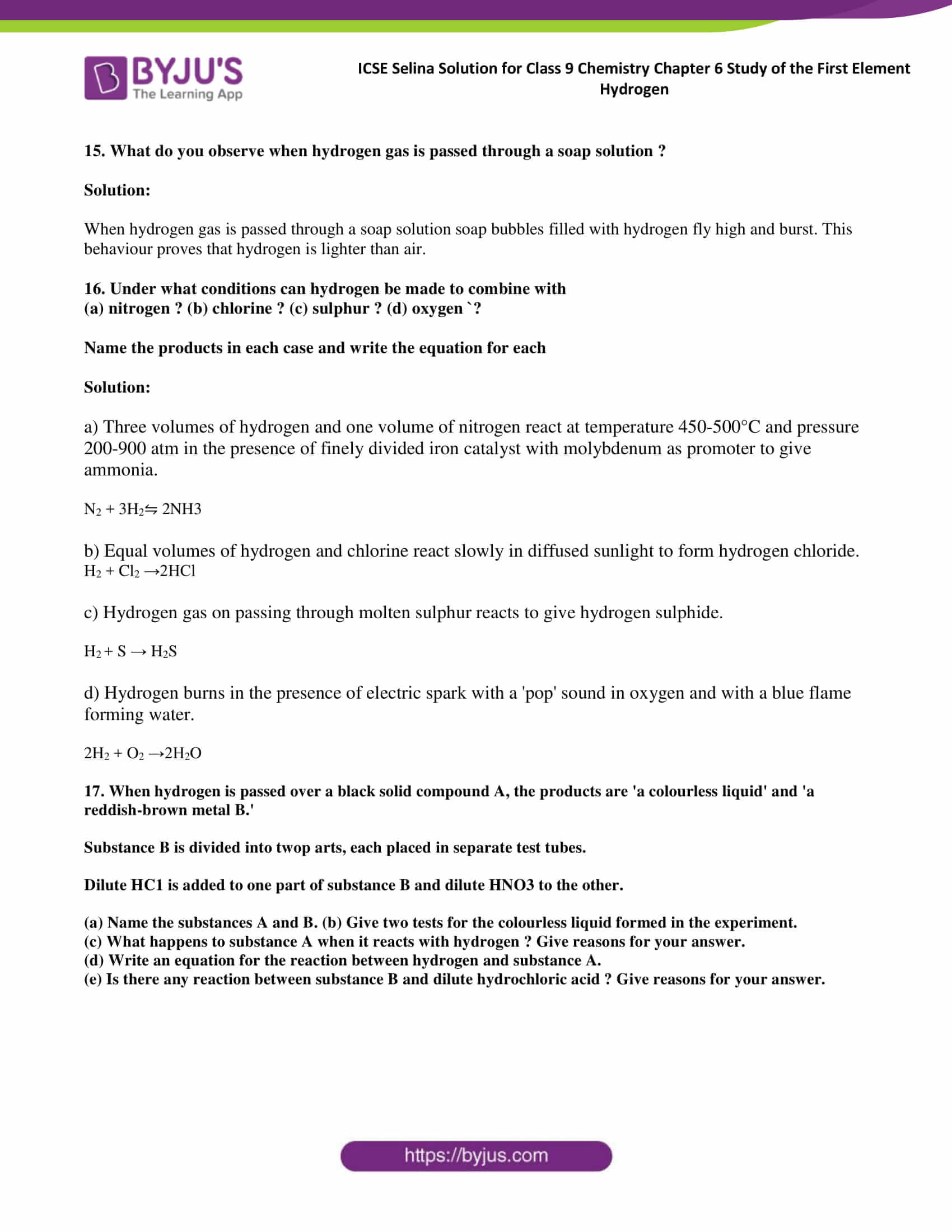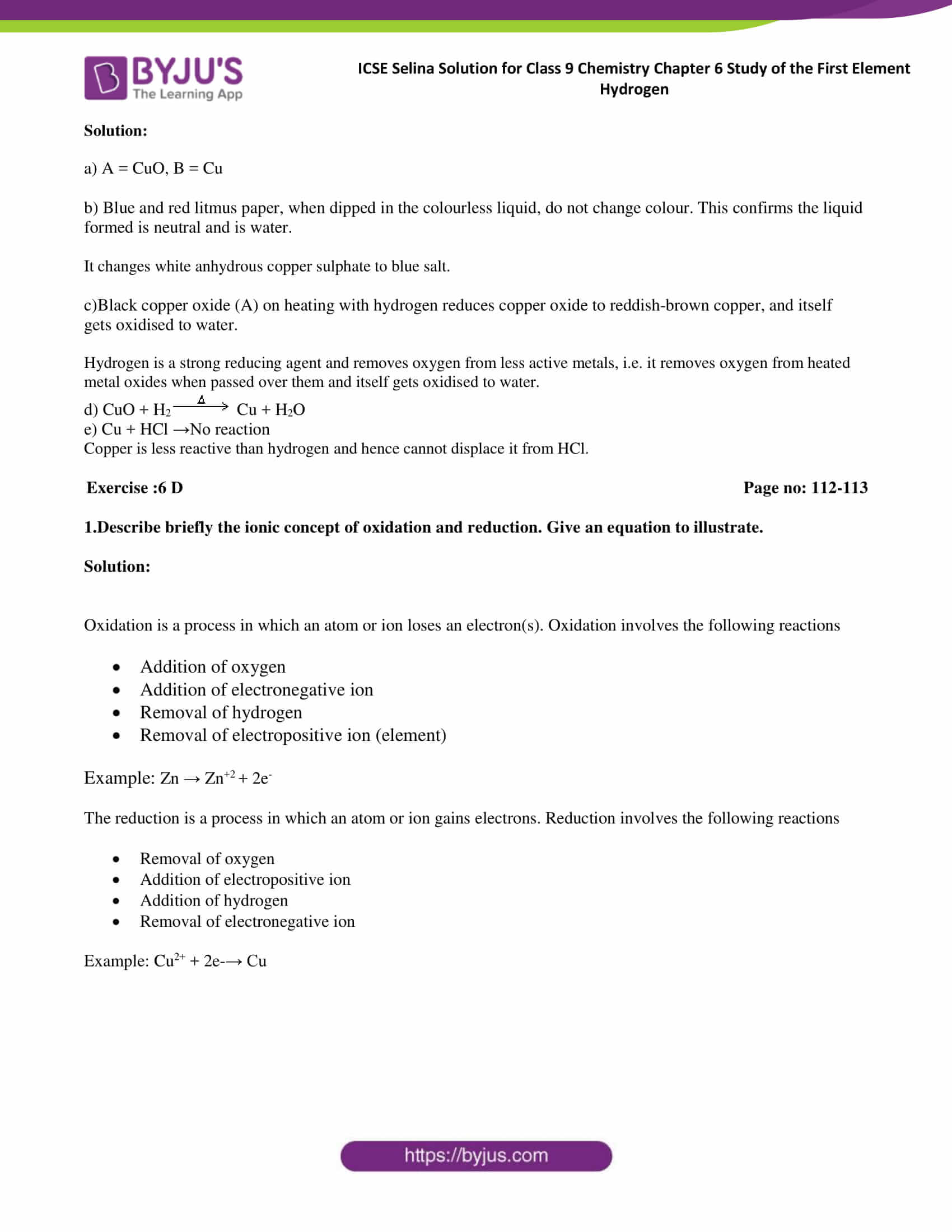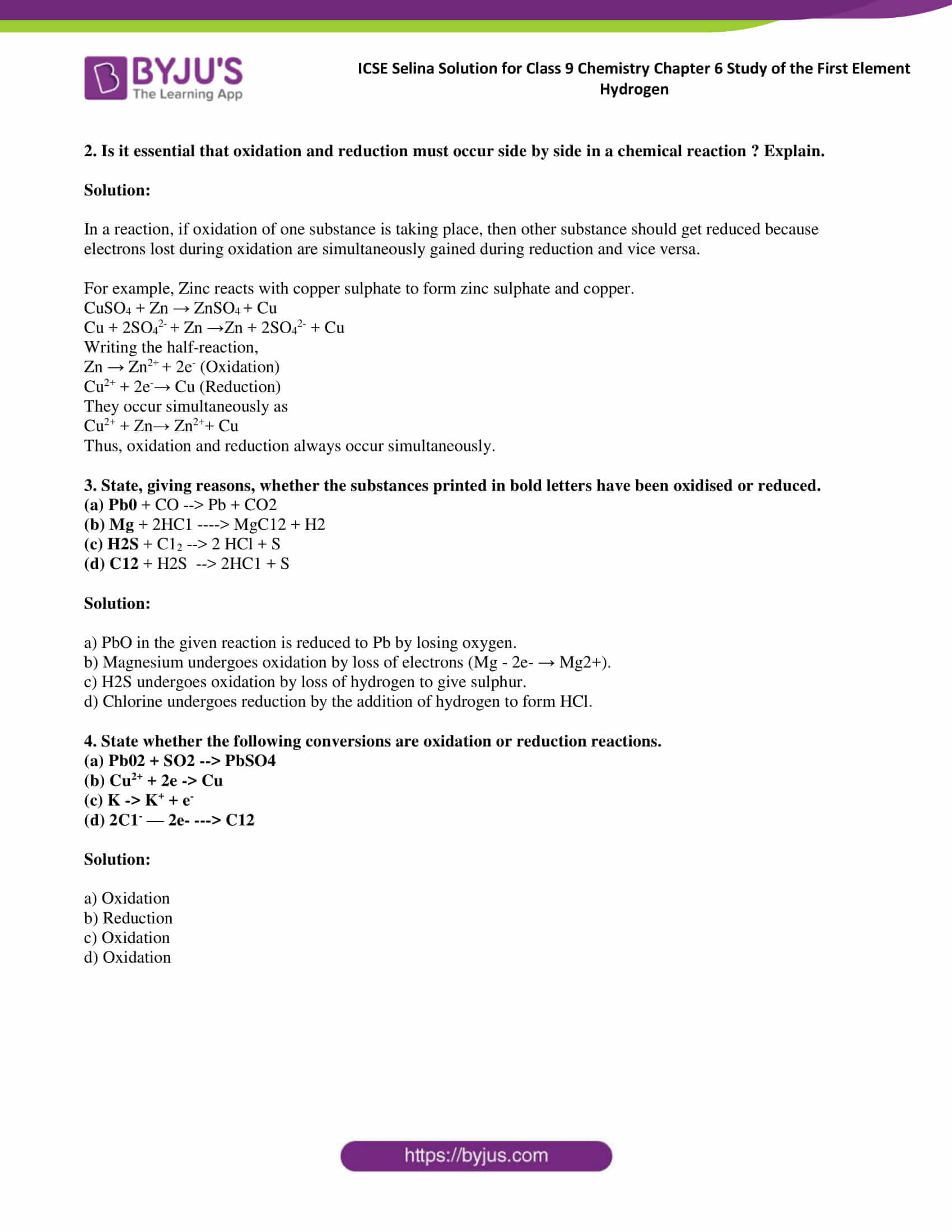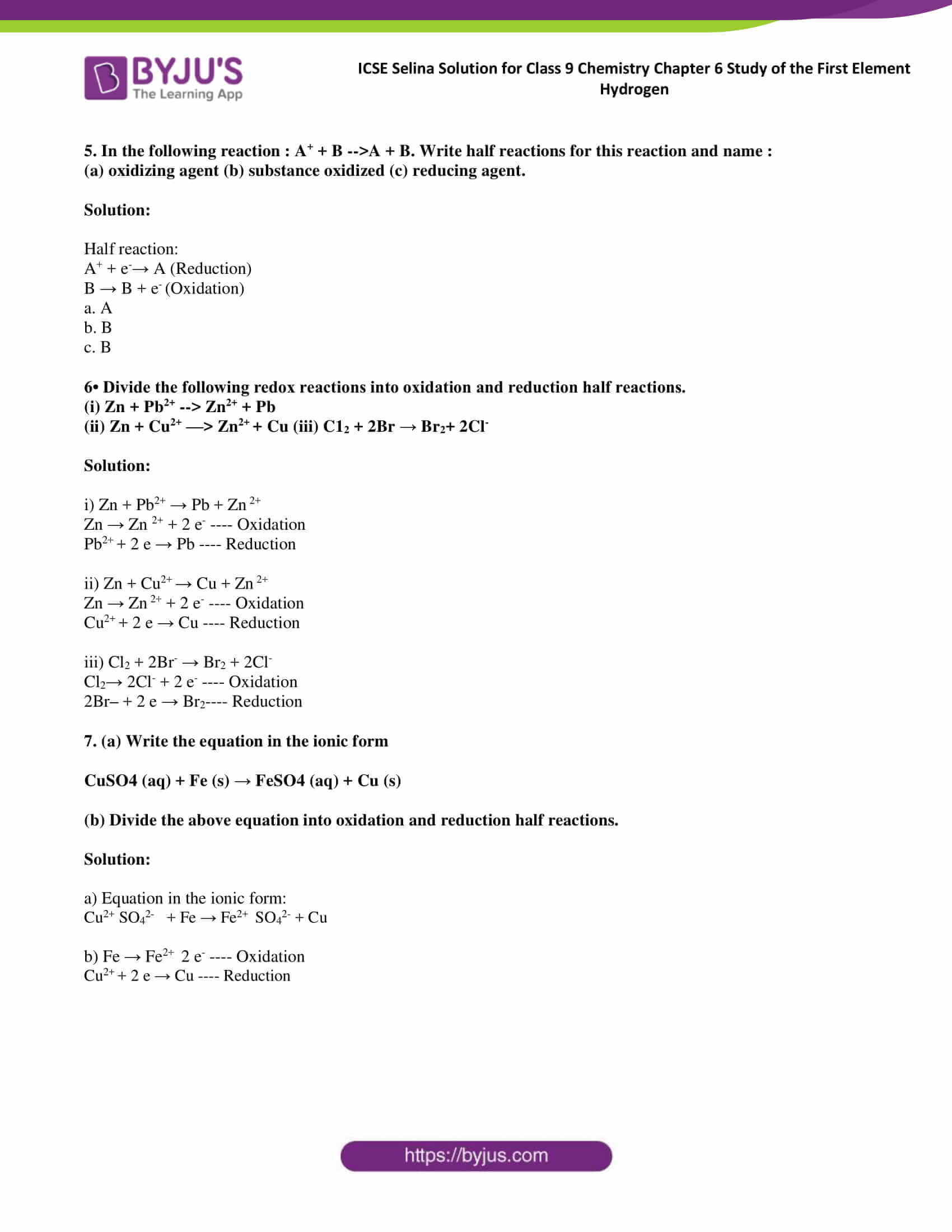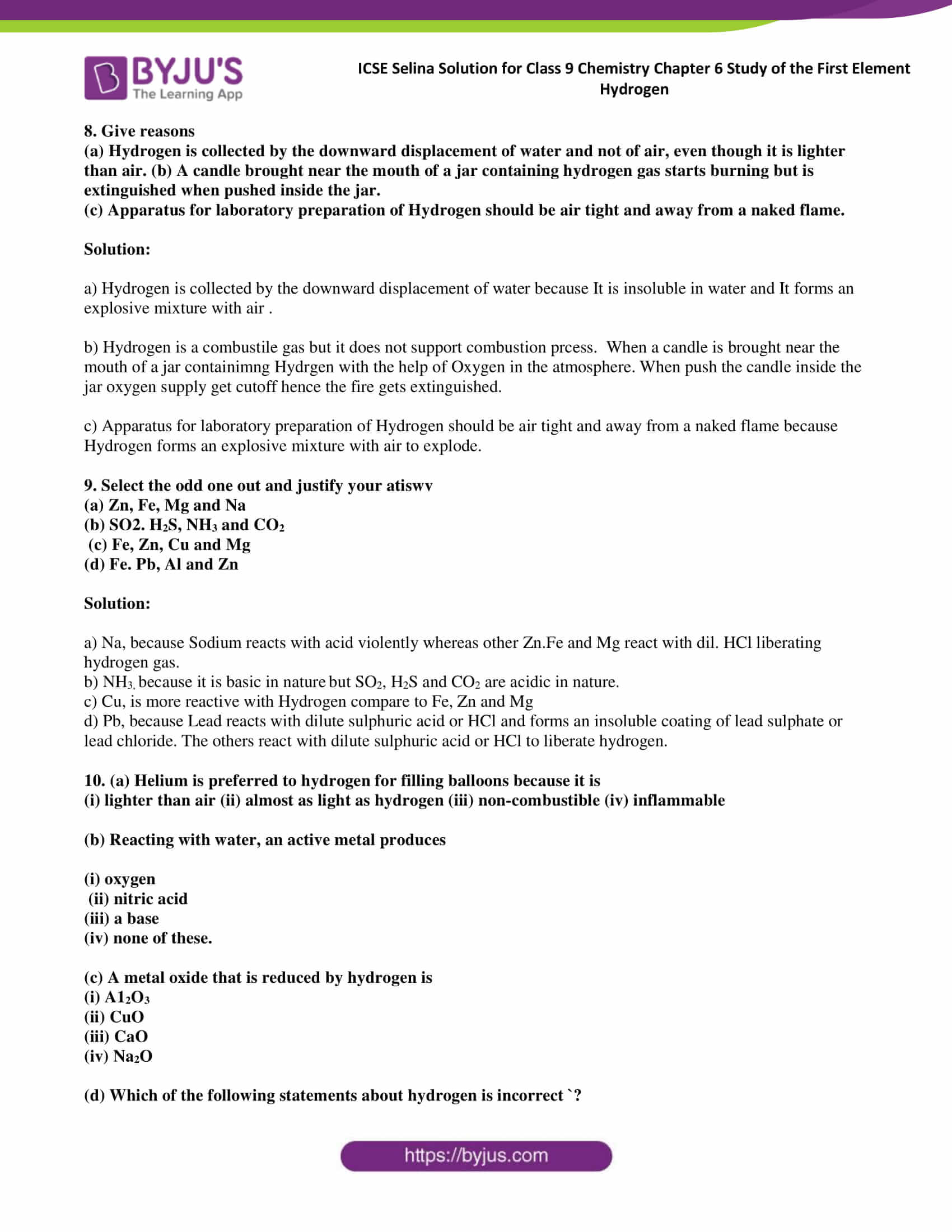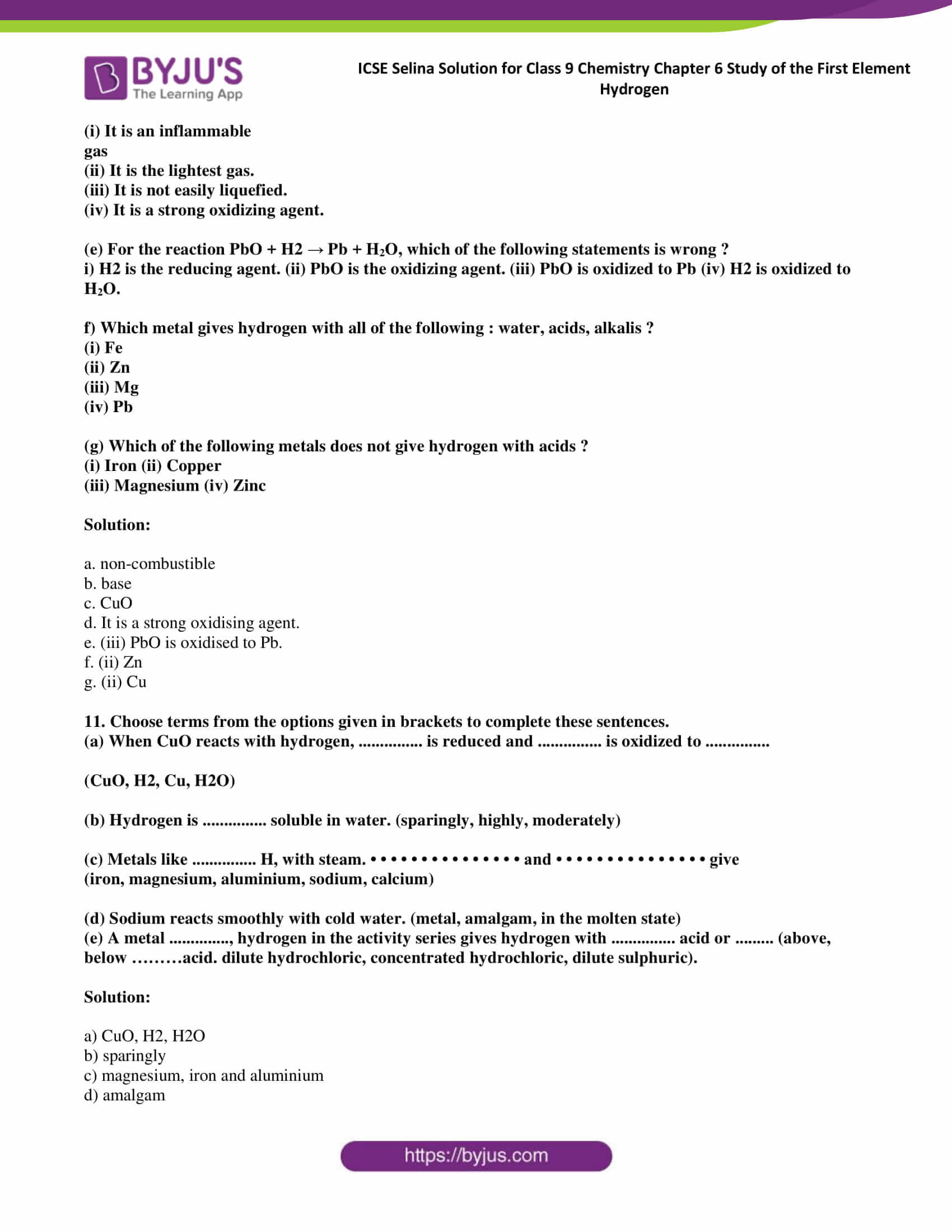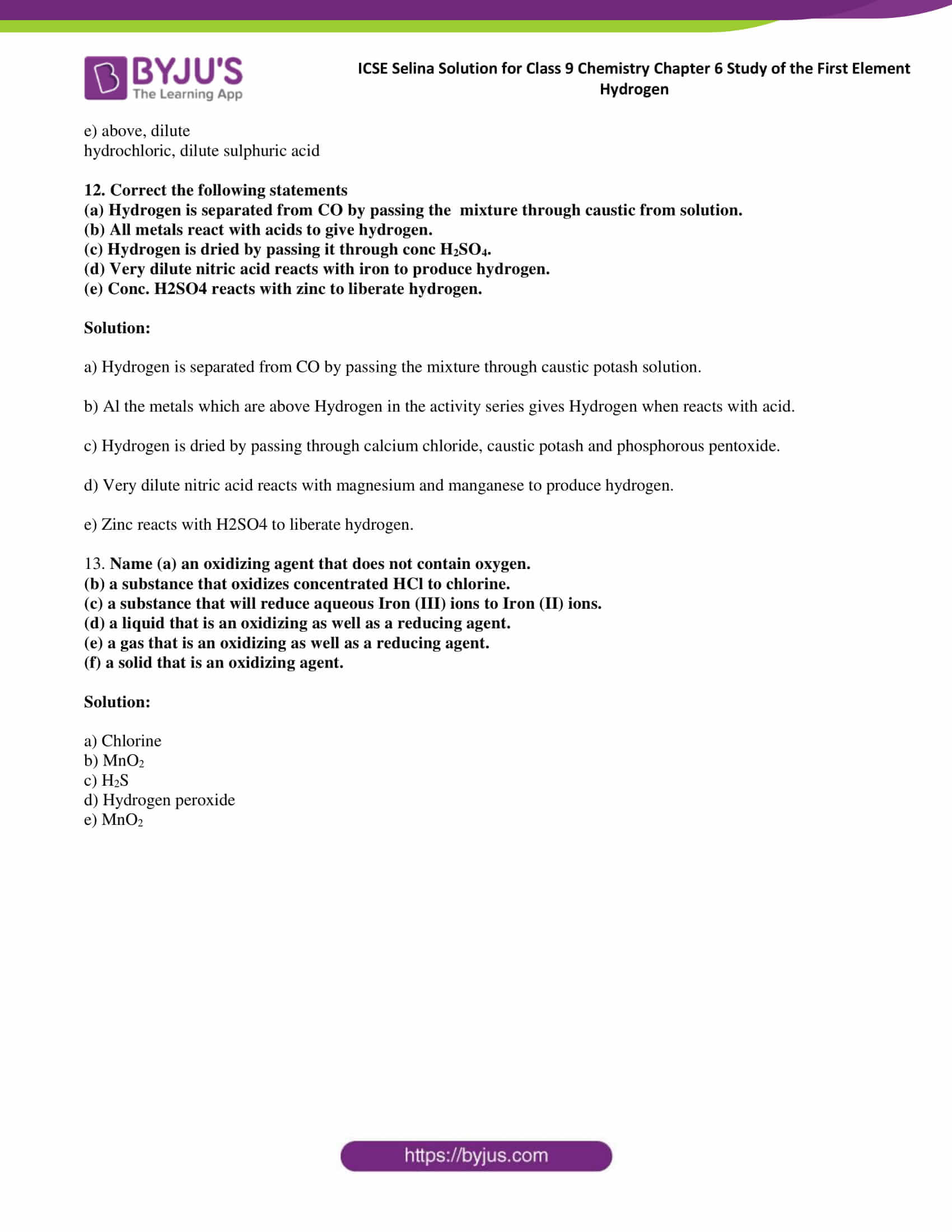### Access Answers of Selina publication ICSE Class 9 Chemistry Chapter 6 The first element Hydrogen

Exercise:6 A

1. Justify the position of hydrogen in the periodic table.

Solution:

Hydrogen has atomic number 1, and it exists in the first group and 1st period of the periodic table. Hence it is the first element of the periodic table.

2. Why does hydrogen show dual nature?

Solution:

Hydrogen resembles the alkali metals of Group IA and the halogens of Group VIIA hence it shows dual nature.

3. Compare hydrogen with alkali metals on the basis of :

i) ion formation (ii) Reducing- power

iii) Reaction with oxygen (iv) Oxide formation

Solution:

a) Hydrogen and Alkali metals can form a cation by the loss of an electron.

b) Both Hydrogen atoms and alkali metals are reducing agents.

c) Both alkali metals and Hydrogen burns in air to form oxides.

d) Hydrogen and Alkali metals can form oxides on reaction with Oxygen.

4 In what respect doses hydrogen differ from

i) Alkali metals ii)Halogens

Solution:

i) Oxides of alkali metals are basic, whereas oxides of Hydrogen is a neutral oxide.

ii) The hydrogen atom has only one shell, but halogens have two or more shells.

5. Give the general group study of hydrogen with reference

i) valence electrons (ii) burning iii) reducing power.

Solution:

i) Hydrogen has 1 valence electron in its orbit.

ii) Hydrogen burns with Oxygen to form oxides of Hydrogen with a pop sound.

2H2 + O2 → 2H2O

iii) Hydrogen acts as a reducing agent.

CuO + H2 → Cu + H2O

6. Why was hydrogen called ‘inflammable air’

Solution:

Hydrogen is highly combustible gas; hence, it is called as inflammable air.

7. State some sources of hydrogen.

Solution:

Hydrogen is found in earth’s crust and in the atmosphere in a free state. It is found in organic compounds in combined state.

8. Compare hydrogen and halogens on the basis of :

i) Physical state ii) ion formation iii) valency (iv) reaction with oxygen

Solution:

a) Both Halogens and Hydrogen are gases

b) Both of them form anions as they are short of 1 electron to take stable confirmation.

c) Both Hydrogen and Halogens have the valency 1.

d) Hydrogen reacts with oxygen to form neutral oxides; Halogen reacts with oxygen to form acidic oxides.

9. Which metal is preferred for the preparation of hydrogen

(i) from water? (ii) from acid?

Solution:

i) For the preparation of Hydrogen from water Reactive metals such as potassium, sodium and calcium are used.

ii) For the preparation of Hydrogen from acid Magnesium, aluminium, zinc and iron metals are used.

10. (i) Write the reaction of steam with a red hot iron.

(ii) Why is this reaction considered a reversible reaction?

(in) How can the reaction proceed continuously?

Solution:

i) 3Fe + 4H2O ⇋ Fe3O4 + 4H2

ii) This reaction is reversible because if hydrogen formed is not removed, then the iron oxide formed is reduced back to iron.

iii) This reaction is reversible. If the hydrogen formed is not removed the iron oxide formed is reduced back to iron. Thus, the reaction can proceed continuously.

11. Why Zinc and aluminium are considered to have a unique nature. Give balanced equations to support your answer.

Solution:

Zinc and aluminium are considered to have a unique nature for the following reasons

i) They can react with acids and alkali to form hydrogen and soluble salt.

Zn + 2NaOH → Na2ZnO2 + H2

2Al + 6NaOH→ 2Na2Al O3 + 3H2

ii) They react with both bases and acids to give salt and water.

ZnO + 2HCl → ZnCl2 + H2O

ZnO + 2NaOH →Na2ZnO2 + H2O

12. Write balanced equations for the following

(i) iron reacts with dil HCl

(ii) Zinc reacts with caustic soda solution

(iii) Lead reacts with potassium hydroxide,

(iv) Aluminium reacts with fused sodium hydroxide

Solution:

i) Fe +2 HC l → FeCl2 + H2

ii) Zn + 2NaOH → Na2ZnO2 + H2

iii) Pb + 2KOH → K2PbO2 + H2

iv) 2Al + 6NaOH→ 2Na2Al O3 + 3H2

13. Write balanced equations and give your observations when the following metals react:

(i) Sodium with cold water

(ii) Calcium with cold water

(iii) Magnesium with boiling water

(iv) Magnesium with steam.

Solution:

i) When Sodium reacts with cold water, Hydrogen gas is evolved with the generation of heat.

2Na + 2H2O →2NaOH + H2

ii) Calcium sinks in water and the reaction is less vigorous.

Ca + 2H2O → Ca(OH)2 + H2

iii) Magnesium reacts with boiling water to produce magnesium hydroxide base liberating hydrogen gas.

Mg + 2H2O →Mg(OH)2 + H2O

iv) Magnesium burns in steam to produce white magnesium oxide and hydrogen gas.

Mg(s)+H2O(g) → MgO(s) + H2(g)

14. (i) Under what conditions iron reacts with water. (ii) Give the balanced equation of the reaction. (iii) What is noticed if the Products are not allowed to escape?

Solution:

i) Iron does not react with water directly. Red hot iron reacts with steam to produce triferric tetra-oxide and hydrogen gas.

ii) 3Fe + 4H2O ⇋ Fe3O4 + 4H2

iii) If the product Hydrogen is not removed then the iron oxide formed is reduced back to iron.

15. From the knowledge of activity series, name a metal which shows the following properties

(i) It reacts readily with cold water

(ii) It displaces hydrogen from hot water

(iii) It displaces hydrogen from dilute HCl

(iv) It forms a base which is insoluble in water.

Solution:

i) Sodium

ii) Magnesium

iii) Zinc

iv) Calcium

16. Complete the following word equations

(a) Sodium hydroxide + zinc -4 hydrogen ………………….

b) Calcium + water —9 calcium hydroxide + ………………….

Solution:

a) Sodium hydroxide + zinc → hydrogen + sodium zincate

b) Calcium + water → calcium hydroxide + hydrogen

Exercise:6 B

1. Hydrogen can be prepared with the metal Zinc by using

(i) acid (ii) alkali (iii ) water

Give an equation in each case.

Solution:

i) Zn + HCl → ZnCl2 + H2

ii) Zn + 2NaOH → Na2ZnO2 + H2

iii) Zn + H2O → ZnO + H2

2. For laboratory preparation of Hydrogen, give the vfollowing (a) materials used

(b) method of collection (c) chemical equation (d) fully labelled diagram.

Solution:

a) Granulated zinc, dilute HCl or dil. H2SO4

b) It is collected by the downward displacement of water.

c) Zn + HCl → ZnCl2 + H2

d)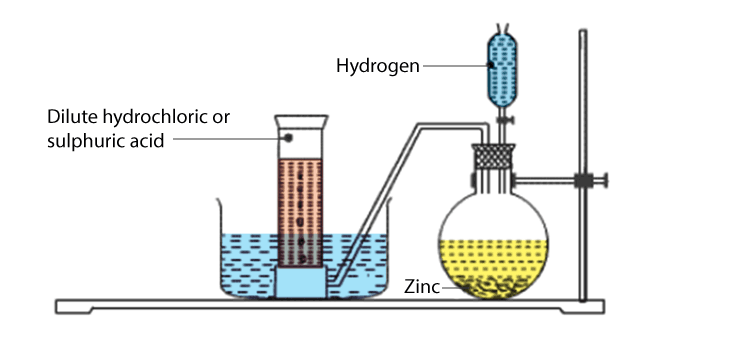3. a) Name the impurities present in hydrogen prepared in the laboratory. (b) How can these impurities be removed?

Solution:

a) Hydrogen sulphide, sulphur dioxide, oxides of nitrogen, phosphine, arsine, carbon dioxide and water vapour are the impurities present in the hydrogen prepared in the laboratory.

b) The impurities can be removed from hydrogen by passing it through

1. Silver nitrate solution to remove arsine and phosphine.

AsH3 + 6AgNO3 → Ag3As + 3AgNO3 + 3HNO3

PH+ 6AgNO→ Ag3P + 3AgNO3 + 3HNO3

2. Lead nitrate solution to remove hydrogen sulphide.

Pb(NO3)2 + H2S → PbS + 2HNO3

3. Caustic potash solution to remove sulphur dioxide, carbon dioxide and oxides of nitrogen.

SO2 + 2KOH → K2SO3 + H2O

CO2 + 2KOH→ K2CO3+ H2O

2NO2 + 2KOH →KNO2 + KNO3 + H2O

4. A drying agent used to dry the gas. Common drying agents such as fused calcium chloride, caustic potash stick and phosphorus pentoxide remove water vapour.

So, the gas is purified and dried and then collected over mercury because mercury does not react with it.

4. Which test should be made before collecting hydrogen in a gas jar?

Solution:

Hydrogen burns with a pop sound, hence Hydrogen should be tested with a burning flame if the burning produces a pop sound it confirms the production of Hydrogen gas.

5. Why is nitric acid not used in the preparation of hydrogen?

Solution:

Nitric acid not used in the preparation of hydrogen because Nitric acid is a powerful oxidising agent, and the oxygen formed due to its decomposition oxidise hydrogen to give water thus defeating the purpose of the reaction.

3Zn + 8HNO3 → 3Zn(NO3)2 + 4H2O + 2NO

6. Why is hot concentrated sulphuric acid not used in the preparation of hydrogen

Solution:

Hot concentrated sulphuric acid not used in the preparation of hydrogen because it will produce sulphur dioxide.

Zn + 2H2SO4 →ZnSO4 + SO2 + 2H2O

7. Hydrogen is manufactured by ‘Bosch Process’.

(a) Give the equations with conditions.

(b) How can you obtain Hydrogen from a mixture of Hydrogen and carbon monoxide?

Solution:

a)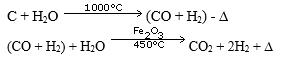b) We can obtain Hydrogen from a mixture of Hydrogen and carbon monoxide by passing the mixture through an ammoniacal cuprous chloride solution in order to dissolve any uncombined carbon monoxide.

CuCl + CO + 2H2O →CuCl.CO.2H2O

8. Give equations to express the reaction between

(a) steam and red hot iron

(b) calcium and water

Solution:

a) 3Fe + 4H2O⇋ Fe3O4 + 4H2

b) Ca + 2H2O ⇋ Ca(OH)2 + H2

9. A small piece of calcium metal is put into a small trough containing water. There is effervescence and white turbidity is formed.

(a) Name the gas formed in the reaction. How would you test the gas?

(b) Write an equation for the reaction.

(c) What do you observe when a few drops of red litmus solution are added to the turbid solution?

Solution:

a) Hydrogen gas, It can be tested by litmus paper as it turn blue from red.

b) Ca + 2H2O → Ca(OH)+ H2

c) The solution turns blue.

10. Thin strips of magnesium, copper and iron are taken. (a) Write down what happens when these metals are treated as follows

(i) Heated in presence of air

(ii) Heated with dil. HCI Added to an aqueous solution of zinc sulphate

(iii) Added to an aqueous solution of Zinc sulphate

(b) Arrange these metals in descending order of reactivity.

Solution:

a) i) When we heat magnesium, copper and iron in presence of air they form respective oxides.

ii) Magnesium and iron react with HCl liberating hydrogen and forming their respective salts.

iii) Magnesium will displace zinc from zinc sulphate solution as magnesium is more reactive than zinc in the activity series of metals.

b) Mg > Fe > Cu

11. Choose the correct option

a) Hydrogen is evolved by the action of cold Dil.HNO3 on

1. Fe B.Cu C.Mg D. Zn

b) Which metal absorbs Hydrogen?

A. Al B. Fe C. Pd D. K

c) The composition of the nucleus of deuterium is

1. 1e and 1P B.1P and 1A c) in and 1e D. 2P and 1e

d) Elements which show unique nature in the preparation of Hydrogen are

1. Na, K, Li B.Mg, Ca, Ba C. Al, Zn, Pb D. Fe, Cu, Ag

Solution:

a) C. Mg

b) C. Pd

c) C. 1 n and 1 e

d) C. Al, Zn, Pb

12. Give the reason for the following

1. Zinc granules are used in the laboratory preparation of Hydrogen.
2. Purified and dry Hydrogen is collected over Mercury.
3. The end of the thistle funnel should be dipped under acid.
4. Dilute Sulphuric acid cannot be replaced by concentrated acid in the preparation of Hydrogen.

Solution:

a) Zinc granules are used in the laboratory preparation of Hydrogen because the impurity present in granulated zinc is copper, whose catalysing effect speeds up the rate of the reaction.

b) Purified and dry Hydrogen is collected over Mercury will not have any impact

c) The end of the thistle funnel should be dipped under acid to prevent the escape of gas from the thistle funnel.

d) Dilute Sulphuric acid cannot be replaced by concentrated acid in the preparation of Hydrogen.

Because it is a strong oxidising agent and it will produce sulphur dioxide.

Exercise:6 C

1. a) Where does Hydrogen occur in free state?

b) How did the name Hydrogen originate?

Solution:

1. Hydrogen occurs in free state n traces in the earth’s crust and atmosphere.
2. The name Hydrogen originated from its ability to form water.

2. Hydrogen can be prepared with the help of cold water. Give a reaction of Hydrogen with:

a) a monovalent metal b) divalent metal

Solution:

a) 2K + 2H2O →2KOH + H2

b) Ca + 2H2O → Ca(OH)2 + H2

3. Which metal is preferred for collecting Hydrogen from

a) Coldwater

b) Hot water

c) Steam

Write a balanced equation for each case.

Solution:

Coldwater: Sodium

2Na + 2H2O → 2NaOH + H2

Hot water: Magnesium

Mg + H2O → MgO + H2

Steam: Aluminium

3Fe + 4H2O → Fe3O4 + H2

4. Hydrogen may be prepared in the laboratory by the action of a metal on an acid.

(a) Which of the metals copper, zinc, magnesium or sodium would be the most suitable?

(b) Which of the acids dilute sulphuric, concentrated sulphuric, dilute nitric and concentrated nitric would you choose?

Explain why you would not use the acids you reject.

(c) How would you modify your apparatus to collect dry hydrogen? Which drying agent would you employ for this purpose?

Solution:

a) Zinc is the most suitable metal to prepare Hydrogen in the laboratory.

b) Dilute sulphuric acid is preferred to prepare Hydrogen in the laboratory because nitric acid in bot concentrated and in diluted form is a strong oxidizing agent. This results in the formation of Oxygen which results in the formation of water. Concentrated sulphuric acid is not used because it will produce sulphur dioxide.

c) The gas is collected by the downward displacement of water. Common drying agents such as fused calcium chloride, caustic potash stick and phosphorus pentoxide remove water vapour.

5. Why are the following metals not used in the lab? preparation of hydrogen?

(a) calcium (b) iron (c) aluminium (d) sodium

Solution:

The answer is (a) calcium because Calcium is expensive.

6. Based on the reactions of water on metals. arrange the following metals in increasing order of reactivity. Iron, sodium magnesium, zinc, calcium.

Solution:

Iron < Zinc < Magnesium < Calcium < Sodium

7. Hydrogen is evolved when dilute HCl reacts with magnesium, but nothing happens in the case of mercury and silver. Explain.

Solution:

Hydrogen is evolved when dilute HCl reacts with magnesium, but nothing happens in the case of mercury and silver because metals which are more reactive than hydrogen can displace it from HCl.

8. Steam can react with a metal and a nonmetal to liberate a product similar to liberate Hydrogen. Give the necessary conditions and equations for the same.

Solution:

Metals with low reactivity react with steam to liberate hydrogen gas. For example, Magnesium reacts with steam produce Magnesium oxide.

Mg + H2O (g) → MgO + H2

9. Hydrogen is obtained by displacement from

a) dilute sulphuric acid

(b) dilute hydrochloric acid

Write equations using zinc and Iron.

Why does not copper show similar behaviour?

Solution:

a) Zn + H2SO4 → ZnSO4 + H2

b) Fe + H2SO4 → FeSO4 + H2

Hydrogen cannot be prepared from metals which are below it in the activity series of metals such as copper because only metals which are more reactive than hydrogen can displace it from acids.

10. Give a reason for the following

a) Though lead is above hydrogen in the activity series, it does not react with dilute hydrochloric acid or dilute sulphuric acid.

(b) Potassium and sodium are not used for reaction with dilute hydrochloric acid or dilute sulphuric acid in the laboratory preparation of hydrogen.

Solution:

a) Lead does not react with hydrochloric acid or dilute sulphuric acid because It forms an insoluble coating of lead sulphate or lead chloride. So, further reaction is prevented.

b) Potassium and sodium are not used for reaction with dilute hydrochloric acid or dilute sulphuric acid in the laboratory preparation of hydrogen because Potassium and sodium react violently with acid.

11. Name two alkalies that can displace hydrogen. Give balanced equations for the same. Why are the metals you have used considered to have unique nature?

Solution:

NaOH and KOH

Zn + 2NaOH → Na2ZnO2 + H2

Zn + 2KOH → K2ZnO2 + H2

Metals such as zinc, lead and aluminium have a unique nature because they react with acids and also with hot alkalis to form hydrogen and soluble salt.

12. Complete and balance the following equations.

a) Na + 2H2O → —– + ——-

b) Ca + 2H2O → —— + ——-

c) Mg + 2H2O → —– + ——–

d) Zn + H2O → ——+ ——–

e) Fe + H2O ⇋ —– + ——-

f) Zn + 2HCl → —— + ——-

g) Al + H2SO4 →—– + ——–

h) Fe +HCl →——+ ——-

i) Zn + 2NaOH → —– + —–

j) Al + 2KOH + 2H2O →—— + ——-

Solution:

a) 2Na + 2H2O → 2NaOH + H2

b) Ca + 2H2O → Ca(OH)2 + H2

c) Mg + 2H2O → Mg(OH)2 + H2

d) Zn + H2O → ZnO + H2

e) 3Fe + 4H2O ⇋Fe3O4 + 4H2

f) Zn + 2HCl → ZnCl2 + H2

g) 2Al + 3H2SO4 →Al2(SO4)3 + 3H2

h) Fe +2HCl →FeCl2 + H2

i) Zn + 2NaOH → Na2ZnO2 + H2

j) 2Al + 2KOH + 2H2O →2KAlO2 + 3H2

13. If the following are kept in closed vessels at over 4000c what would happen to them ?

(a) iron filings and steam,

(b) hydrogen and magnetic oxide of iron ?

Solution:

a) Iron oxide is formed with the evolution of hydrogen gas.

b) Heated magnetic oxide of iron gets reduced by hydrogen.

14. a) A metal in the powdered form reacts very slowly with the boiling water, but it decomposes steam. Name the metal.

1. Write a balanced equation for the reaction of the named metal with (i) boiling water (ii) steam.

Solution:

a) Magnesium

b) Mg + 2H2O → Mg(OH)2 + H2

Mg + H2O →MgO + H2

15. What do you observe when hydrogen gas is passed through a soap solution?

Solution:

When hydrogen gas is passed through a soap solution soap bubbles filled with hydrogen fly high and burst. This behaviour proves that hydrogen is lighter than air.

16. Under what conditions can hydrogen be made to combine with

(a) nitrogen ? (b) chlorine ? (c) sulphur ? (d) oxygen ?

Name the products in each case and write the equation for each

Solution:

a) Three volumes of hydrogen and one volume of nitrogen react at temperature 450-500°C and pressure 200-900 atm in the presence of finely divided iron catalyst with molybdenum as a promoter to give ammonia.

N2 + 3H2⇋ 2NH3

b) Equal volumes of hydrogen and chlorine react slowly in diffused sunlight to form hydrogen chloride.

H2 + Cl2 →2HCl

c) Hydrogen gas on passing through molten sulphur reacts to give hydrogen sulphide.

H+ S → H2S

d) Hydrogen burns in the presence of electric spark with a ‘pop’ sound in oxygen and with a blue flame forming water.

2H2 + O2 →2H2O

17. When hydrogen is passed over a black solid compound A, the products are ‘a colourless liquid’ and ‘a reddish-brown metal B.’

Substance B is divided into two parts, each placed in separate test tubes.

Dilute HC1 is added to one part of substance B and dilute HNO3 to the other.

(a) Name the substances A and B. (b) Give two tests for the colourless liquid formed in the experiment.

(c) What happens to substance A when it reacts with hydrogen ? Give reasons for your answer.

(d) Write an equation for the reaction between hydrogen and substance A.

(e) Is there any reaction between substance B and dilute hydrochloric acid ? Give reasons for your answer.

Solution:

a) A = CuO, B = Cu

b) Blue and red litmus paper, when dipped in the colourless liquid, do not change colour. This confirms the liquid formed is neutral and is water.

It changes white anhydrous copper sulphate to blue salt.

c)Black copper oxide (A) on heating with hydrogen reduces copper oxide to reddish-brown copper, and itself gets oxidised to water.

Hydrogen is a strong reducing agent and removes oxygen from less active metals, i.e. it removes oxygen from heated metal oxides when passed over them and itself gets oxidised to water.

d) CuO + H2

$$\begin{array}{l}\overset{\bigtriangleup }{\rightarrow}\end{array}$$
Cu + H2O

e) Cu + HCl →No reaction

Copper is less reactive than hydrogen and hence cannot displace it from HCl.

exercise:6 D

1. Describe briefly the ionic concept of oxidation and reduction. Give an equation to illustrate.

Solution:

Oxidation is a process in which an atom or ion loses an electron(s). Oxidation involves the following reactions

• Removal of hydrogen
• Removal of electropositive ion (element)

Example: Zn → Zn+2 + 2e

The reduction is a process in which an atom or ion gains electrons. Reduction involves the following reactions

• Removal of oxygen
• Removal of electronegative ion

Example: Cu2+ + 2e-→ Cu

2. Is it essential that oxidation and reduction must occur side by side in a chemical reaction? Explain.

Solution:

In a reaction, if oxidation of one substance is taking place, then other substance should get reduced because electrons lost during oxidation are simultaneously gained during reduction and vice versa.

For example, Zinc reacts with copper sulphate to form zinc sulphate and copper.

CuSO4 + Zn → ZnSO+ Cu

Cu + 2SO42- + Zn →Zn + 2SO42- + Cu

Writing the half-reaction,

Zn → Zn2+ + 2e (Oxidation)

Cu2+ + 2e→ Cu (Reduction)

They occur simultaneously as

Cu2+ + Zn→ Zn2++ Cu

Thus, oxidation and reduction always occur simultaneously.

3. State, giving reasons, whether the substances printed in bold letters have been oxidised or reduced.

(a) PbO + CO → Pb + CO2

(b) Mg + 2HC1 → MgC12 + H2

(c) H2S + C12 → 2 HCl + S

(d) C12 + H2S → 2HC1 + S

Solution:

a) PbO in the given reaction is reduced to Pb by losing oxygen.

b) Magnesium undergoes oxidation by loss of electrons (Mg – 2e- → Mg2+).

c) H2S undergoes oxidation by loss of hydrogen to give sulphur.

d) Chlorine undergoes reduction by the addition of hydrogen to form HCl.

4. State whether the following conversions are oxidation or reduction reactions.

(a) PbO2 + SO2 → PbSO4

(b) Cu2+ + 2e → Cu

(c) K → K+ + e

(d) 2C1 — 2e- → C12

Solution:

a) Oxidation

b) Reduction

c) Oxidation

d) Oxidation

5. In the following reaction: A+ + B →A + B. Write half-reactions for this reaction and name :

(a) oxidizing agent (b) substance oxidized (c) reducing agent.

Solution:

Half reaction:

A+ + e→ A (Reduction)

B → B + e– (Oxidation)

a. A

b. B

c. B

6• Divide the following redox reactions into oxidation and reduction half reactions.

(i) Zn + Pb2+ → Zn2+ + Pb

(ii) Zn + Cu2+ → Zn2+ + Cu (iii) C12 + 2Br → Br2+ 2Cl

Solution:

i) Zn + Pb2+ → Pb + Zn 2+

Zn → Zn 2+ + 2 e —- Oxidation

Pb2+ + 2 e → Pb —- Reduction

ii) Zn + Cu2+ → Cu + Zn 2+

Zn → Zn 2+ + 2 e —- Oxidation

Cu2+ + 2 e → Cu —- Reduction

iii) Cl2 + 2Br → Br2 + 2Cl–

Cl2→ 2Cl + 2 e —- Oxidation

2Br + 2 e → Br2—- Reduction

7. (a) Write the equation in the ionic form

CuSO4 (aq) + Fe (s) → FeSO4 (aq) + Cu (s)

(b) Divide the above equation into oxidation and reduction half reactions.

Solution:

a) The equation in the ionic form:

Cu2+ SO42-   + Fe → Fe2+  SO42- + Cu

b) Fe → Fe2+  2 e —- Oxidation

Cu2+ + 2 e → Cu —- Reduction

8. Give reasons

(a) Hydrogen is collected by the downward displacement of water and not of air, even though it is lighter than air. (b) A candle brought near the mouth of a jar containing hydrogen gas starts burning but is extinguished when pushed inside the jar.

(c) Apparatus for laboratory preparation of Hydrogen should be airtight and away from a naked flame.

Solution:

a) Hydrogen is collected by the downward displacement of water because It is insoluble in water and It forms an explosive mixture with air.

b) Hydrogen is a combustible gas but it does not support the combustion process. When a candle is brought near the mouth of a jar containing Hydrogen with the help of Oxygen in the atmosphere. When pushing the candle inside the jar oxygen supply get cut off hence the fire gets extinguished.

c) Apparatus for laboratory preparation of Hydrogen should be airtight and away from a naked flame because Hydrogen forms an explosive mixture with air to explode.

(a) Zn, Fe, Mg and Na

(b) SO2. H2S, NH3 and CO2

(c) Fe, Zn, Cu and Mg

(d) Fe. Pb, Al and Zn

Solution:

a) Na, because Sodium reacts with acid violently whereas other Zn. Fe and Mg react with dil. HCl liberating hydrogen gas.

b) NH3, because it is basic in nature but SO2, H2S and CO2 are acidic in nature.

c) Cu, is more reactive with Hydrogen compare to Fe, Zn and Mg

d) Pb because Lead reacts with dilute sulphuric acid or HCl and forms an insoluble coating of lead sulphate or lead chloride. The others react with dilute sulphuric acid or HCl to liberate hydrogen.

10. (a) Helium is preferred to hydrogen for filling balloons because it is

(i) lighter than air (ii) almost as light as hydrogen (iii) non-combustible (iv) inflammable

(b) Reacting with water, an active metal produces

(i) oxygen

(ii) nitric acid

(iii) a base

(iv) none of these.

(c) A metal oxide that is reduced by hydrogen is

(i) A12O3

(ii) CuO

(iii) CaO

(iv) Na2O

(d) Which of the following statements about hydrogen is incorrect ?

(i) It is an inflammable gas

(ii) It is the lightest gas.

(iii) It is not easily liquefied.

(iv) It is a strong oxidizing agent.

(e) For the reaction PbO + H2 → Pb + H2O, which of the following statements is wrong?

i) H2 is the reducing agent. (ii) PbO is the oxidizing agent. (iii) PbO is oxidized to Pb (iv) H2 is oxidized to H2O.

f) Which metal gives hydrogen with all of the following: water, acids, alkalis?

(i) Fe

(ii) Zn

(iii) Mg

(iv) Pb

(g) Which of the following metals does not give hydrogen with acids?

(i) Iron (ii) Copper

(iii) Magnesium (iv) Zinc

Solution:

a. non-combustible

b. base

c. CuO

d. It is a strong oxidising agent.

e. (iii) PbO is oxidised to Pb.

f. (ii) Zn

g. (ii) Cu

11. Choose terms from the options given in brackets to complete these sentences.

(a) When CuO reacts with hydrogen, …………… is reduced and …………… is oxidized to ……………

(CuO, H2, Cu, H2O)

(b) Hydrogen is …………… soluble in water. (sparingly, highly, moderately)

(c) Metals like …………… H, with steam. • • • • • • • • • • • • • • • and • • • • • • • • • • • • • • • give

(iron, magnesium, aluminium, sodium, calcium)

(d) Sodium reacts smoothly with cold water. (metal, amalgam, in the molten state)

(e) A metal ………….., hydrogen in the activity series gives hydrogen with …………… acid or ……… (above, below ………acid. dilute hydrochloric, concentrated hydrochloric, dilute sulphuric).

Solution:

a) CuO, H2, H2O

b) sparingly

c) magnesium, iron and aluminium

d) amalgam

e) above, dilute hydrochloric, dilute sulphuric acid

12. Correct the following statements

(a) Hydrogen is separated from CO by passing the mixture through caustic from solution.

(b) All metals react with acids to give hydrogen.

(c) Hydrogen is dried by passing it through conc H2SO4.

(d) Very dilute nitric acid reacts with iron to produce hydrogen.

(e) Conc. H2SO4 reacts with zinc to liberate hydrogen.

Solution:

a) Hydrogen is separated from CO by passing the mixture through caustic potash solution.

b) Al the metals which are above Hydrogen in the activity series gives Hydrogen when reacts with acid.

c) Hydrogen is dried by passing through calcium chloride, caustic potash and phosphorous pentoxide.

d) Very dilute nitric acid reacts with magnesium and manganese to produce hydrogen.

e) Zinc reacts with H2SO4 to liberate hydrogen.

13. Name (a) an oxidizing agent that does not contain oxygen.

(b) a substance that oxidizes concentrated HCl to chlorine.

(c) a substance that will reduce aqueous Iron (III) ions to Iron (II) ions.

(d) a liquid that is an oxidizing as well as a reducing agent.

(e) a gas that is an oxidizing as well as a reducing agent.

(f) a solid that is an oxidizing agent.

Solution:

a) Chlorine

b) MnO2

c) H2S

d) Hydrogen peroxide

e) MnO2

## Important topics covered in ICSE Selina Solution for Class 9 Chemistry Chapter 6

• Position of Hydrogen in Periodic Table
• Similarities Between Hydrogen and Alkali metals
• Similarities Between Hydrogen and Halogens
• Discovery of Hydrogen
• Occurrence of Hydrogen
• Preparation of Hydrogen
• Application of activity series in the preparation of Hydrogen
• Laboratory preparation of Hydrogen
• Manufacture of Hydrogen
• Properties of Hydrogen
• Uses of Hydrogen
• Oxidation and Reduction

BYJU’S provides best study materials, important questions, ICSE Books, solved papers and previous year question papers for all classes and all the subjects of ICSE syllabus. For more information download the BYJU’S learning App.

## Frequently Asked Questions on Study of the first element Hydrogen

Q1

### Why does hydrogen show dual nature?

Hydrogen resembles the alkali metals of Group IA and the halogens of Group VIIA hence it shows dual nature.

Q2

### State some sources of hydrogen?

Hydrogen is found in earth’s crust and in the atmosphere in a free state. It is found in organic compounds in combined state.

Q3

### Which metal is preferred for the preparation of hydrogen from water?

For the preparation of Hydrogen from water Reactive metals such as potassium, sodium and calcium are used.

Q4

### Which test should be made before collecting hydrogen in a gas jar?

Hydrogen burns with a pop sound, hence Hydrogen should be tested with a burning flame if the burning produces a pop sound it confirms the production of Hydrogen gas.

Q5

### Which metal is preferred for the preparation of hydrogen from acid?

For the preparation of Hydrogen from acid Magnesium, aluminium, zinc and iron metals are used.

Q6

### Why is hot concentrated sulphuric acid not used in the preparation of hydrogen?

Hot concentrated sulphuric acid not used in the preparation of hydrogen because it will produce sulphur dioxide.
Zn + 2H2SO4 →ZnSO4 + SO2 + 2H2O

Q7

### What do you observe when hydrogen gas is passed through a soap solution?

When hydrogen gas is passed through a soap solution soap bubbles filled with hydrogen fly high and burst. This behaviour proves that hydrogen is lighter than air.